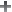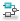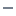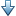Main Content

## Weighted Variance Goal

### Purpose

Frequency-weighted limit on noise impact on specified output signals for tuning with Control System Tuner.

### Description

Weighted Variance Goal limits the noise impact on the outputs of the frequency-weighted transfer function WL(s)H(s)WR(s), where H(s) is the transfer function between inputs and outputs you specify. WL(s) and WR(s) are weighting functions you can use to model a noise spectrum or emphasize particular frequency bands. Thus, you can use Weighted Variance Goal to tune the system response to stochastic inputs with a nonuniform spectrum such as colored noise or wind gusts.

Weighted Variance minimizes the response to noise at the inputs by minimizing the H2 norm of the frequency-weighted transfer function. The H2 norm measures:

• The total energy of the impulse response, for deterministic inputs to the transfer function.

• The square root of the output variance for a unit-variance white-noise input, for stochastic inputs to the transfer function. Equivalently, the H2 norm measures the root-mean-square of the output for such input.

#### Creation

In the Tuning tab of Control System Tuner, select New Goal > Frequency-weighted variance attenuation to create a Weighted Variance Goal.

#### Command-Line Equivalent

When tuning control systems at the command line, use `TuningGoal.WeightedVariance` to specify a weighted gain goal.

### I/O Transfer Selection

Use this section of the dialog box to specify noise input locations and response outputs. Also specify any locations at which to open loops for evaluating the tuning goal.

• Specify stochastic inputs

Select one or more signal locations in your model as noise inputs. To constrain a SISO response, select a single-valued input signal. For example, to constrain the gain from a location named `'u'` to a location named `'y'`, clickAdd signal to list and select `'u'`. To constrain the noise amplification of a MIMO response, select multiple signals or a vector-valued signal.

• Specify stochastic outputs

Select one or more signal locations in your model as outputs for computing response to the noise inputs. To constrain a SISO response, select a single-valued output signal. For example, to constrain the gain from a location named `'u'` to a location named `'y'`, clickAdd signal to list and select `'y'`. To constrain the noise amplification of a MIMO response, select multiple signals or a vector-valued signal.

• Compute output variance with the following loops open

Select one or more signal locations in your model at which to open a feedback loop for the purpose of evaluating this tuning goal. The tuning goal is evaluated against the open-loop configuration created by opening feedback loops at the locations you identify. For example, to evaluate the tuning goal with an opening at a location named `'x'`, clickAdd signal to list and select `'x'`.

Tip

To highlight any selected signal in the Simulink® model, click. To remove a signal from the input or output list, click. When you have selected multiple signals, you can reorder them usingand. For more information on how to specify signal locations for a tuning goal, see Specify Goals for Interactive Tuning.

### Weights

Use the Left weight WL and Right weight WR text boxes to specify the frequency-weighting functions for the tuning goal.

WL provides the weighting for the output channels of H(s), and WR provides the weighting for the input channels.

You can specify scalar weights or frequency-dependent weighting. To specify a frequency-dependent weighting, use a numeric LTI model whose magnitude represents the desired weighting as a function of frequency. For example, enter `tf(1,[1 0.01])` to specify a high weight at low frequencies that rolls off above 0.01 rad/s. To limit the response to a nonuniform noise distribution, enter as WR an LTI model whose magnitude represents the noise spectrum.

If the tuning goal constrains a MIMO transfer function, scalar or SISO weighting functions automatically expand to any input or output dimension. You can specify different weights for each channel by specifying MIMO weighting functions. The dimensions H(s) must be commensurate with the dimensions of WL and WR. For example, if the constrained transfer function has two inputs, you can specify `diag([1 10])` as WR.

If you are tuning in discrete time, you can specify the weighting functions as discrete-time models with the same sampling time as you use for tuning. If you specify the weighting functions in continuous time, the tuning software discretizes them. Specifying the weighting functions in discrete time gives you more control over the weighting functions near the Nyquist frequency.

### Options

Use this section of the dialog box to specify additional characteristics of the weighted variance goal.

• Apply goal to

Use this option when tuning multiple models at once, such as an array of models obtained by linearizing a Simulink model at different operating points or block-parameter values. By default, active tuning goals are enforced for all models. To enforce a tuning requirement for a subset of models in an array, select Only Models. Then, enter the array indices of the models for which the goal is enforced. For example, suppose you want to apply the tuning goal to the second, third, and fourth models in a model array. To restrict enforcement of the requirement, enter `2:4` in the Only Models text box.

For more information about tuning for multiple models, see Robust Tuning Approaches (Robust Control Toolbox).

### Tips

• When you use this requirement to tune a control system, Control System Tuner attempts to enforce zero feedthrough (D = 0) on the transfer that the requirement constrains. Zero feedthrough is imposed because the H2 norm, and therefore the value of the tuning goal (see Algorithms), is infinite for continuous-time systems with nonzero feedthrough.

Control System Tuner enforces zero feedthrough by fixing to zero all tunable parameters that contribute to the feedthrough term. Control System Tuner returns an error when fixing these tunable parameters is insufficient to enforce zero feedthrough. In such cases, you must modify the requirement or the control structure, or manually fix some tunable parameters of your system to values that eliminate the feedthrough term.

When the constrained transfer function has several tunable blocks in series, the software’s approach of zeroing all parameters that contribute to the overall feedthrough might be conservative. In that case, it is sufficient to zero the feedthrough term of one of the blocks. If you want to control which block has feedthrough fixed to zero, you can manually fix the feedthrough of the tuned block of your choice.

To fix parameters of tunable blocks to specified values, see View and Change Block Parameterization in Control System Tuner.

• This tuning goal also imposes an implicit stability constraint on the weighted closed-loop transfer function between the specified inputs to outputs, evaluated with loops opened at the specified loop-opening locations. The dynamics affected by this implicit constraint are the stabilized dynamics for this tuning goal. The Minimum decay rate and Maximum natural frequency tuning options control the lower and upper bounds on these implicitly constrained dynamics. If the optimization fails to meet the default bounds, or if the default bounds conflict with other requirements, on the Tuning tab, use Tuning Options to change the defaults.

### Algorithms

When you tune a control system, the software converts each tuning goal into a normalized scalar value f(x). Here, x is the vector of free (tunable) parameters in the control system. The software then adjusts the parameter values to minimize f(x) or to drive f(x) below 1 if the tuning goal is a hard constraint.

For Weighted Variance Goal, f(x) is given by:

`$f\left(x\right)={‖WL\text{\hspace{0.17em}}H\left(s,x\right)\text{\hspace{0.17em}}WR‖}_{2}.$`

H(s,x) is the closed-loop transfer function between the specified inputs and outputs, evaluated with parameter values x. ${‖\text{\hspace{0.17em}}\cdot \text{\hspace{0.17em}}‖}_{2}$ denotes the H2 norm (see `norm`).

For tuning discrete-time control systems, f(x) is given by:

`$f\left(x\right)=\frac{1}{\sqrt{{T}_{s}}}{‖WL\left(z\right)\text{\hspace{0.17em}}H\left(z,x\right)\text{\hspace{0.17em}}WR\left(z\right)‖}_{2}.$`

Ts is the sample time of the discrete-time transfer function H(z,x).

## SupportGet trial now